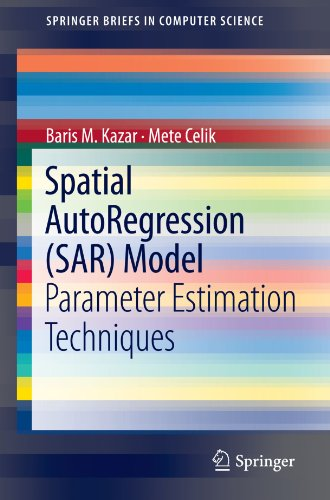# Spatial AutoRegression (SAR) Model: Parameter Estimation Techniques

• Length: 83 pages
• Edition: 2012
• Publisher:
• Publication Date: 2012-03-31
• ISBN-10: 1461418410
• ISBN-13: 9781461418412
Description

Explosive growth in the size of spatial databases has highlighted the need for spatial data mining techniques to mine the interesting but implicit spatial patterns within these large databases. This book explores computational structure of the exact and approximate spatial autoregression (SAR) model solutions. Estimation of the parameters of the SAR model using Maximum Likelihood (ML) theory is computationally very expensive because of the need to compute the logarithm of the determinant (log-det) of a large matrix in the log-likelihood function. The second part of the book introduces theory on SAR model solutions. The third part of the book applies parallel processing techniques to the exact SAR model solutions. Parallel formulations of the SAR model parameter estimation procedure based on ML theory are probed using data parallelism with load-balancing techniques. Although this parallel implementation showed scalability up to eight processors, the exact SAR model solution still suffers from high computational complexity and memory requirements. These limitations have led the book to investigate serial and parallel approximate solutions for SAR model parameter estimation. In the fourth and fifth parts of the book, two candidate approximate-semi-sparse solutions of the SAR model based on Taylor’s Series expansion and Chebyshev Polynomials are presented. Experiments show that the differences between exact and approximate SAR parameter estimates have no significant effect on the prediction accuracy. In the last part of the book, we developed a new ML based approximate SAR model solution and its variants in the next part of the thesis. The new approximate SAR model solution is called the Gauss-Lanczos approximated SAR model solution. We algebraically rank the error of the Chebyshev Polynomial approximation, Taylor’s Series approximation and the Gauss-Lanczos approximation to the solution of the SAR model and its variants. In other words, we established a novel relationship between the error in the log-det term, which is the approximated term in the concentrated log-likelihood function and the error in estimating the SAR parameter for all of the approximate SAR model solutions.